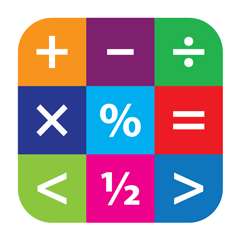# What is the number riddle

A number has three digits. The sum of the three digits equals 36 times of the number. Seven times the left digit plus 9 is equal to 5 times the sum of the two other digits. 8 times the second digit minus 9 is equal to the sum of the first and third.

What is the number ?324

with the conditions specified in the question, we get three equations

1. 7x+9=5y+5z
2. 8y-9=x+z
3.36x+36y+36z=100x+10y+z

by solving these equations we get x=3,y=2,z=4

Looking at the exact text this problem isn’t solvable.
The problem says: “The sum of the three digits equals 36 times of the number“.
The sum of the digits is at most 27 (3 times 9) which is always less than 36 times the lowest 3 digit number (36 * 100).

When the condition is “The number equals 36 times the sum of the three digits” then you get the solution dyj mentioned.
This condition only has two numbers that meets the condition: 324 and 648.
648 does not meet the other two conditions. So we only needed one of these two conditions to find 324 as the answer.